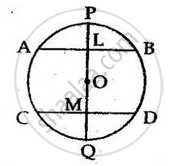Share

Books Shortlist

# The Line Joining the Mid-points of Two Chords of a Circle Passes Through Its Centre. Prove that the Chords Are Parallel. - ICSE Class 10 - Mathematics

ConceptChord Properties - There is One and Only One Circle that Passes Through Three Given Points Not in a Straight Line

#### Question

The line joining the mid-points of two chords of a circle passes through its centre. Prove that the
chords are parallel.

#### SolutionGiven : AB and CD are the two chords of a circle with centre O.
L and M are the midpoints of AB and CD and O lies in the line joining ML
To prove: AB ∥ CD
Proof: AB and CD are two chords of a circle with centre O.
Line LOM bisects them at L and M
Then, OL ⊥ AB
And, OM ⊥ CD
∴ ∠ALM = ∠LMD = 90°
But they are alternate angles
∴ AB ∥ CD.

Is there an error in this question or solution?

#### APPEARS IN

Solution The Line Joining the Mid-points of Two Chords of a Circle Passes Through Its Centre. Prove that the Chords Are Parallel. Concept: Chord Properties - There is One and Only One Circle that Passes Through Three Given Points Not in a Straight Line.
S